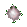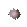Generate a Sine Wave Graph from a Circle - Trigonometry Help - Game Tips:

- When you ride a ferris wheel, your vertical positions compared to the centre of the wheel
form a sine wave pattern based on the rotation angle of the ferris wheel.

- The equation y = A sin(T)   represents a set of ordered pairs of the form (T,y)
with y representing vertical height and T representing the angle of rotation.

- When graphed on a horizontal T-axis and vertical y-axis, the curve represented by y = A sin(T)
is a sine wave having vertical amplitude = |A| and horizontal wavelength = 2(Pi).

- In the ferris wheel example, A is the maximum vertical distance above the centre of the wheel.
The amplitude of the wave is based on the radius of the ferris wheel. In this game r=1 unit.

- Wavelength refers to how long it takes for a pattern to repeat itself. Eg. a crest + a trough .
The wavelength of the wave is based on one complete wheel rotation = 360 degrees.

- A rotation of 360 degrees is also described in Math as an angle of 2(Pi) radians.
This means that one wavelength of y = A sin(T) is approximately 2(3.14159) = 6.3 units long.
[Radian measure can be based on a circle's Circumference = 2(Pi)r, where Pi=3.14159 approx.]

- The basic sine wave y= sin(T) has amplitude 1 unit and an approximate wavelength of 6.3 units.

- See the wheel spin & get your Score Report after you have made 6 choices.
- Your Game Score is reduced by the number of star hits.

- To slow the game speed repeat tap/click on the word Slider.
- To increase the game speed repeat tap/click on the word Math.
- Speed can also be adjusted with a keyboard's - and + keys.

- Refresh/Reload the web page to restart the game.